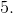# High School Math : How to find the length of a side of a polygon

## Example Questions

### Example Question #1 : How To Find The Length Of A Side Of A Polygon

If the area of a regular octagon is 160 and the apothem is 8, what is the side length?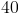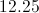Explanation:

To find the side length from the area of an octagon and the apothem we must use the area of a polygon which is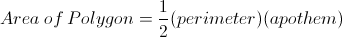First plug in our numbers for area and the apothem to get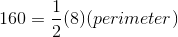Then multiply to get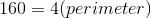Then divide both sides by 4 to get the perimeter of the figure.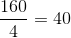When we have the perimeter of a regular polygon, to find the side length we must divide by the number of sides of the polygon, in this case 8.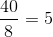After dividing we find the side length is